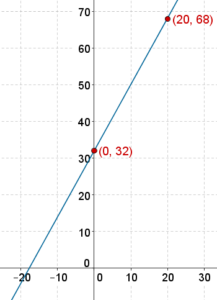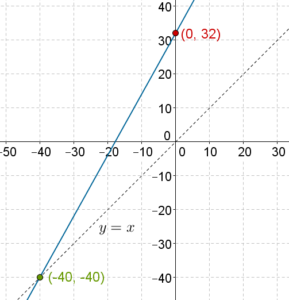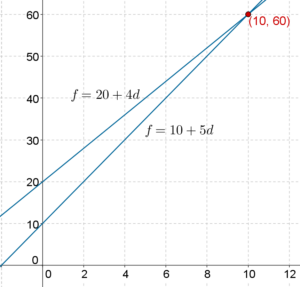# Practical Applications of Linear Equations

Practical Applications of Linear Equations

Linear equations arise in a lot of practical situations. For example, consider the two widely used temperature scales: Celsius and Fahrenheit. To convert from the Fahrenheit scale to the Celsius scale, the following relation is used:

$F = \left( {\frac{9}{5}} \right)C + 32$

Note carefully that this is a linear equation in the two variables F and C. Let us plot the graph for this linear equation using two particular solutions:

 $C =$ $$0$$ $$20$$ $F =$ $$32$$ $$68$$ $$sol$$ $\left( {0,32}\right)$ $\left( {20,68}\right)$

The graph is plotted below:Using this graph, we can convert easily from Celsius to Fahrenheit and vice-versa. For example, we note that $$P\left( {30,86} \right)$$ lies on the graph, which means that when $$C = 30$$, then $$F = 86$$, that is, $${30^0}C$$ is $${86^0}F$$. Similarly, we see that $$Q\left( {35,95} \right)$$ lies on the graph, that is, $${35^0}C$$ is $${95^0}F$$.

We also note that the line intersects the y-axis at roughly $$\left( { - 17.7,0} \right)$$, which means that $${0^0}F$$ is approximately $$- {17.7^0}C$$.

Is there a temperature whose numerical value is the same in both scales? To determine such a temperature, we need to determine a point on the line both of whose coordinates are the same, that is, a point for which

x-coordinate = y-coordinate

To do this, we draw the line $$y = x$$ on the Celsius-Fahrenheit graph. Wherever the line $$y = x$$ intersects the Celsius-Fahrenheit line, the point obtained will have the same x and y coordinates (can you see why?):We see that the intersection point is $$\left( { - 40, - 40} \right)$$. Thus, $$- {40^0}C$$ when converted to Fahrenheit scale is $$- {40^0}F$$. This temperature has the same numerical value on both the scales.

Example 1: In a certain city, there are two kinds of taxis available: black and yellow. Black taxis charge a down-payment of Rs. 20 and a per-km fare of Rs. 4. Yellow taxis charge a down-payment of Rs. 10 and per-km fare of Rs. 5.

I. Use $$f$$ to denote the total fare (in rupees) and $$d$$ to denote the distance covered (in km). Construct linear equations related $$f$$ and $$d$$ for the black and yellow taxis.

II. Draw graphs for these equations.

III. Under what conditions is a black taxi cheaper than a yellow taxi?

Solution:

I. For the black taxi, we have $$f = 20 + 4d$$, while for the yellow taxi, we have $$f = 10 + 5d$$.

II. The two linear equations are plotted on the same axes below (the scale for the vertical axis is different than the horizontal axis):Note that the two lines intersect at the point $$\left( {10,60} \right)$$, that is, at $$d = 10,\,f = 60$$.

III. For distances less than 10 km, the line for the black taxi lies above the yellow taxi (that is, fares for the black taxi will be higher). When $$d = 10$$ km, the fares for the two taxis will be the same (Rs. 60). When $$d > 10$$ km, the line for the black taxi goes below that of the yellow taxi. Thus, for distances greater than 10 km, a black taxi will be cheaper.

Linear Equations
Linear Equations
grade 9 | Questions Set 2
Linear Equations
grade 9 | Questions Set 1
Linear Equations
More Important Topics
Numbers
Algebra
Geometry
Measurement
Money
Data
Trigonometry
Calculus
More Important Topics
Numbers
Algebra
Geometry
Measurement
Money
Data
Trigonometry
Calculus